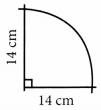# MCQ Questions for Class 7 Maths Chapter 11 Perimeter and Area with Answers

We have compiled the NCERT MCQ Questions for Class 7 Maths Chapter 11 Perimeter and Area with Answers Pdf free download covering the entire syllabus. Practice MCQ Questions for Class 7 Maths with Answers on a daily basis and score well in exams. Refer to the Perimeter and Area Class 7 MCQs Questions with Answers here along with a detailed explanation.

## Perimeter and Area Class 7 MCQs Questions with Answers

Choose the correct option.

Question 1.
The area of a square plot is 1600 m². The side of the plot is
(a) 40 m
(b) 80 m
(c) 120 m
(d) 160 m

Question 2.
The perimeter of a rectangle is 30 m. Its length is 10 m its breadth is …………
(a) 5 m
(b) 10 m
(c) 15 m
(d) 3 m

Question 3.
The area of a parallelogram of base 5 cm and height 3.2 cm is …………..
(a) 8 cm²
(b) 12 cm²
(c) 16 cm²
(d) 20 cm²

Question 4.
Find the area of the following parallelogram(a) 6 cm²
(b) 8 cm²
(c) 12 cm²
(d) 24 cm²

Question 5.
Find AD in the following figure, if area of ∆ABC is 6 cm².(a) 3 cm
(b) 4 cm
(c) 5 cm
(d) 2.4 cm

Question 6.
Length of a rectangle is 8 cm and diagonal is 10 cm. Its area is
(a) 96 cm²
(b) 80 cm²
(c) 60 cm²
(d) 48 cm²

Question 7.
Perimeter of a semi circle is
(a) $$\frac {1}{2}$$ πr²
(b) πr + d
(c) πr
(d) 3πr²

Question 8.
One hectare is ……….
(a) 1000 m
(b) 10000 m
(c) 1000 m²
(d) 10000 m²

Question 9.
The perimeter of the given figure is(a) 50 cm
(b) 36 cm
(c) 25 cm
(d) 28 cm

Question 10.
The diameter of a circle is 7 cm, its area is ……….
(a) 154 cm²
(b) 38.5 cm²
(c) 22 cm²
(d) 11 cm²

Question 11.
The area between two concentric circles of radius R and r is:
(a) 2πR² – 2πr²
(b) π(R² – r²)(R² + r²)
(c) π(R + r)(R – r)
(d) 2π(R + r)(R – r)

Answer: (c) π(R + r)(R – r)

Question 12.
The area of the shaded region is:(a) 12 cm²
(b) 24 cm²
(c) 36 cm²
(d) 18 cm²

Fill in the blanks

Question 1.
If the side of a square is 13 cm, then its area is ……………… cm².

Question 2.
If the side of an equilateral triangle is 5 cm. Its perimeter is ………………… cm.

Question 3.
The area of a triangle is 330 cm². If its altitude is 11 cm, then its base = …………….. cm.

Question 4.
The base and the corresponding height of a triangle are 5 cm and 4.2 cm respectively. Its area is ……………… cm².

Question 5.
The radius of a circle is 7 cm. The area of the semicircle is ……………….

Question 6.
The base and the corresponding height of a triangle are 5 cm and 4.2 cm respectively. Its area is ………………… cm².

Question 7.
If the side of a square is 10 cm, then its area is ………………………. cm².

Question 8.
The perimeter of a square is numerically equal to its area. The side of the square is ……………………

Question 9.
The perimeter of a rectangle is 50 cm. If its length is 15 cm, then its breadth is …………………… cm.

Question 10.
If the perimeter of an equilateral triangle is 3 cm. Its area is ……………….. cm².

Answer: $$\frac {√3}{4}$$

Question 11.
1 hectare = …………………. m².

Question 12.
The diameter of a circle is 14 cm. Its area is …………………. cm².

Question 13.
The diameter of a circle is 2 cm. Its area is ……………………. cm².

Answer: $$\frac {22}{7}$$

Question 14.
If radius of a circle is 1 cm, then the circumference of its semi-circle is …………………… cm.

Answer: $$\frac {36}{7}$$
Answer: $$\frac {44}{7}$$cm²